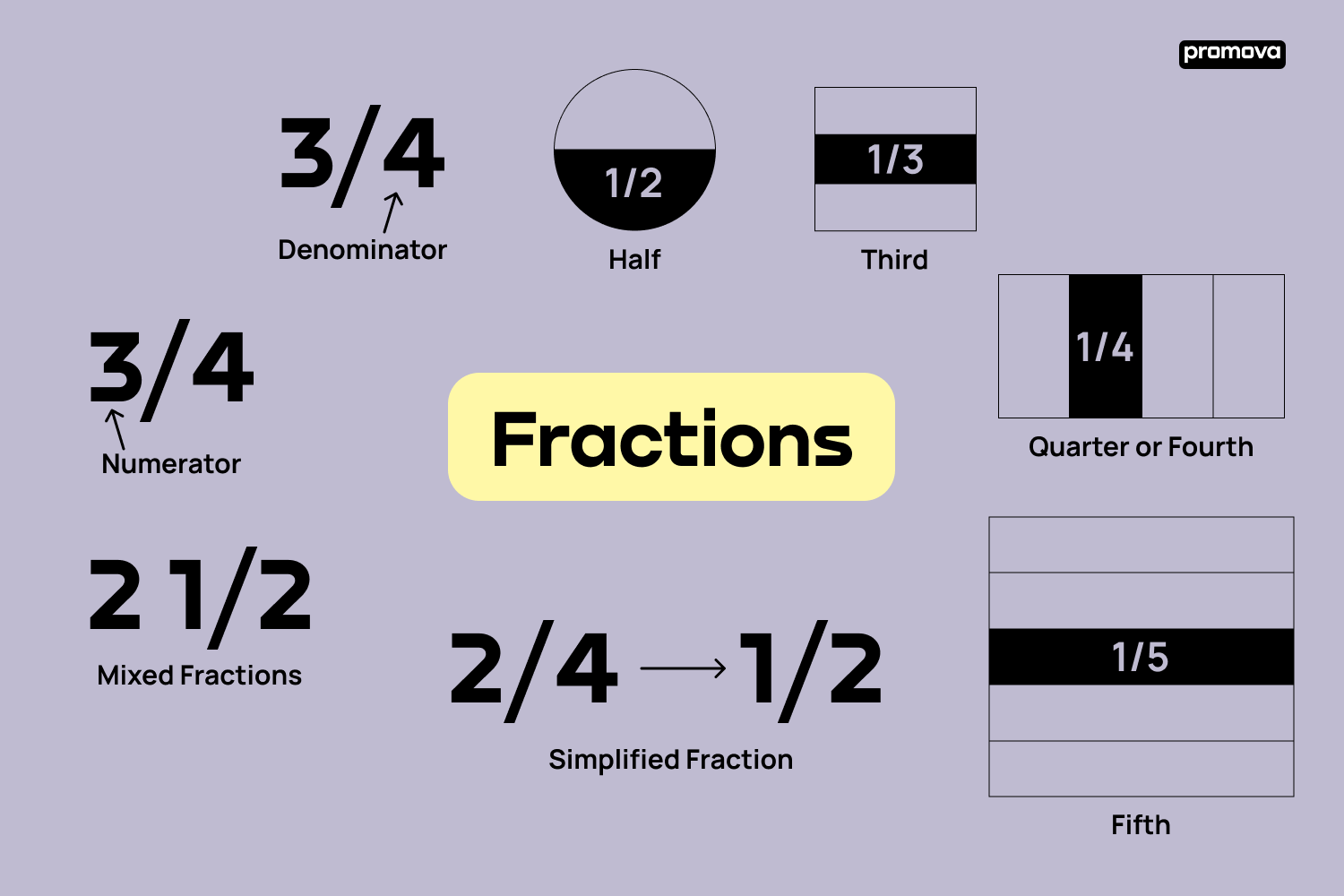# Fractions Vocabulary in English

reviewed byIryna Andrus

## Contents

Today, you will learn how to name fractions in the English language. If you're a language learner striving to understand the vocabulary of fractions, then this guide will be helpful.## What is a Fraction?

In mathematics, a fraction is a fundamental concept used to represent a part of a whole or any number of equal parts. It's formed by two numbers separated by a slash (/) or a horizontal line. Fractions allow us to work with amounts that are not whole numbers, bridging the gap between integers.

## Basic Terms Related to Fractions

Here are some basic terms related to fractions.

• Numerator: This is the top number in a fraction which signifies the number of parts we have. Example: In 3/4, 3 is the numerator.
• Denominator: This is the bottom number in a fraction showing how many equal parts the whole is divided into. Example: In 3/4, 4 is the denominator.

Before we proceed, remember each fraction word has a specific meaning, so understanding them will help you better articulate mathematical ideas.

## Basic Fractions

Basic fractions refer to a set of common and fundamental fractional numbers that are frequently used in mathematics and everyday situations. These fractions represent simple divisions of a whole into equal parts. Basic fractions include those with denominators (the bottom number) from 2 to 10, as they are the most commonly encountered fractions.

• Half: 1/2, pronounced as "one-half."
• Third: 1/3, pronounced as "one-third."
• Quarter or Fourth: 1/4, pronounced as "one quarter" or "one-fourth".
• Fifth: 1/5, pronounced as "one-fifth."

The name of the fraction corresponds to the denominator, which is why 1/2 is called "one-half," 1/3 is "one-third," and so on.

15

## Types of Fractions

• Common Fraction/Proper Fraction: When the numerator is less than the denominator. Example: 3/4, pronounced as "three-fourths."
• Top-heavy fraction/Improper Fraction: When the numerator is greater than or equal to the denominator. Example: 7/3, pronounced as "seven thirds."
• Equivalent Fraction: Different fractions that represent the same value. Example: 1/2 is equivalent to 2/4.
• Simplified Fraction: A fraction that has been reduced to its simplest form. Example: 2/4 simplified is 1/2.
• Unit Fraction: A fraction where the numerator is 1 and the denominator is a positive integer. Example: 1/3, pronounced as "one-third."
• Like Fractions: Fractions with the same denominator. Example: 2/5 and 3/5 are like fractions.
• Unlike Fractions: Fractions with different denominators. Example: 1/2 and 2/3 are unlike fractions.
• Mixed Fractions: A number composed of a whole number and a fraction. Example: 2 1/2, pronounced as "two and one-half."

These types of fractions add a new layer of depth to your understanding of fraction vocabulary.

## Conclusion

Understanding these fraction vocabulary words will help you confidently navigate through any discussion involving fractions in English. Remember, learning is a continuous process, and every new word adds to your language skills.

Try Promova to improve your language level